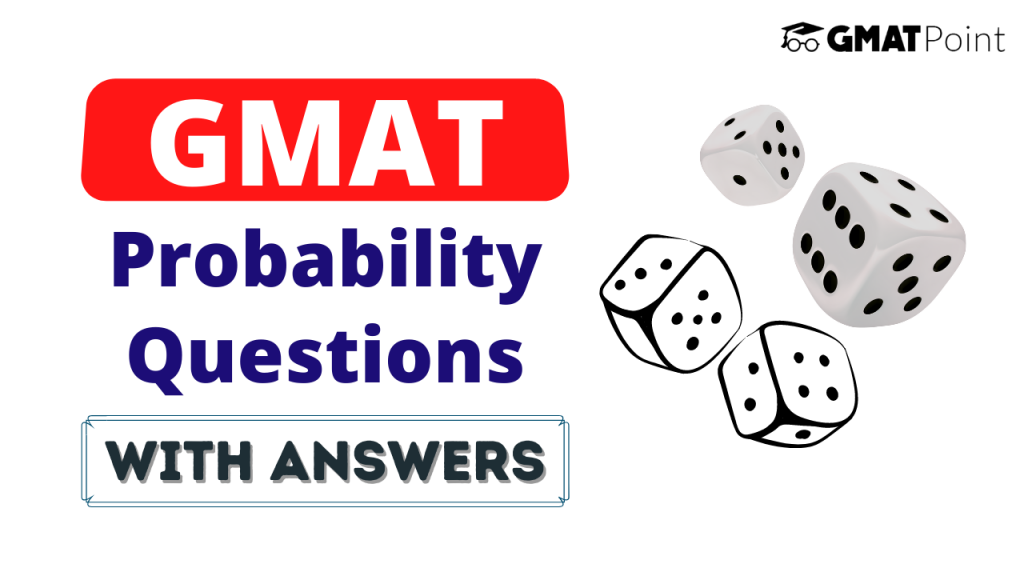# GMAT Probability Questions With Answers# GMAT Probability Questions With Answers

Probability questions constitute a major portion of the Quant section of the GMAT. These questions can come under both Problem Solving and Data Sufficiency types. Probability has a number of concepts and formulae in it. In this article, we will be looking into –

1. GMAT Probability Questions with Answers

## Examples – GMAT Probability Questions with Answers and explanations

### Question 1:

What is the probability of getting a sum of at least 8 in the roll of two fair dice?

### Approach:

In probability, we need to calculate the total number of outcomes and the total number of favourable outcomes individually.

Total number of outcomes = 6 x 6 = 36

Favourable outcomes:

1. Sum = 8 -> {2,6}, {6,2}, {3,5}, {5,3}, {4,4}
2. Sum = 9 -> {3,6}, {6,3}, {4,5}, {5,4}
3. Sum = 10 -> {4,6}, {6,4}, {5.5}
4. Sum = 11 -> {5,6}, {6,5}
5. Sum = 12 -> {6,6}

Sum = 15

Probablity = 15/36 = 5/12

### Question 2:

If the probability of getting a head if we throw a biased coin is double the probability of getting a tail, what is the probability of getting one head and one tail when we throw the coin twice?

### Approach:

Probability(H) = 2 Probability(T)

-> P(H) + P(T) = 1

-> P(H) = 2/3

-> P(T) = 1/3

P(1 H and 1 T) = P(HT) + P(TH) = 2 x 2/3 x 1/3 = 4/9

### Question 3:

If we choose two consecutive alphabets from A to Z, what is the probability that one of them is a vowel?

### Approach:

The number of ways of choosing two consecutive alphabets = 26 – 1 = 25.

The number of ways such that one of them is a vowel is 9 -> AB, DE, EF, HI, IJ, NO, OP, TU, UV

Hence, the probability = 9/25

### Note:

Though these examples provide a good sense of what type of GMAT Probability questions you can expect, in no way do they represent the exhaustive list of concepts required for the Quantitative section of the GMAT.

### Tips to keep in mind:

1. Try to take some time out from the Problem-Solving Probability questions so that you can use that time to solve the tricky Data Sufficiency Probability questions.
2. Do not get stuck in a question for long. If you find yourself trapped in a question for long, take a guess and move on.
3. Read the conditions/constraints(if any) carefully.
4. Look out for negation words. For example: Which of the following are NOT possible values of x?
5. Some questions can be solved faster by the use of options. Make sure you don’t solve these questions in a conventional way.

You can check out the Free GMAT Daily Targets on our platform.

Also, check out the Free GMAT Verbal Tests and Quant Tests.

If you are starting your GMAT preparation from scratch, do check out GMATPOINT.